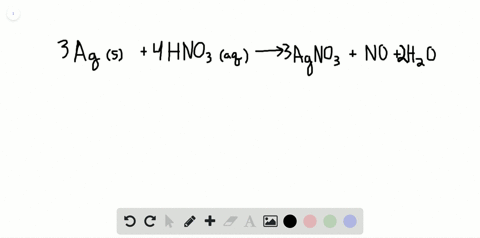### Silver can be separated from gold because silver …

01:30Problem 14

Indicate what type, or types, of reaction each of the following represents:
(a) $\mathrm{H}_{2} \mathrm{O}(g)+\mathrm{C}(s) \longrightarrow \mathrm{CO}(g)+\mathrm{H}_{2}(g)$
(b) $2 \mathrm{KClO}_{3}(s) \longrightarrow 2 \mathrm{KCl}(s)+3 \mathrm{O}_{2}(g)$
(c) Al(OH) $_{3}(a q)+3 \mathrm{HCl}(a q) \longrightarrow \mathrm{AlCl}_{3}(a q)+3 \mathrm{H}_{2} \mathrm{O}(l)$
(d) $\mathrm{Pb}\left(\mathrm{NO}_{3}\right)_{2}(a q)+\mathrm{H}_{2} \mathrm{SO}_{4}(a q) \longrightarrow \mathrm{PbSO}_{4}(s)+2 \mathrm{HNO}_{3}(a q)$

(a) redox reaction
(b) redox reaction
(c) acid-base reaction
(d) precipitation reaction

## Discussion

You must be signed in to discuss.

## Video Transcript

this question. Ask house to indicate the types off the reaction. Let's see. Question eight order reacts with carbon to produce carbon monoxide under on hydrogen. I guess this is a replacement reaction. As you can see the hydrogen, your water is replaced with carbon on. This is also a Redox reaction. As you can see, the the water is a oxidizing agent, and the carbon is the reducing agent on DDE. Question. Be potassium, potassium chlorate, decomposed decomposes, too. Potassium Call Reg and gases oxygen. This is a redox reaction, as you can see the the oxidation number off oxygen you potassium chlorate. It's different from the oxy off sedition number off the oxygen your oxygen gas on the question C Questions. See, is a typical acid based reaction, because in the in a product, there is information on water, and the reactions is why I say that One base on the question deep Question D. Let's see the product. There is the formation off, lad. A soffit solely the last saw fit. So this is a precipitation reaction. So